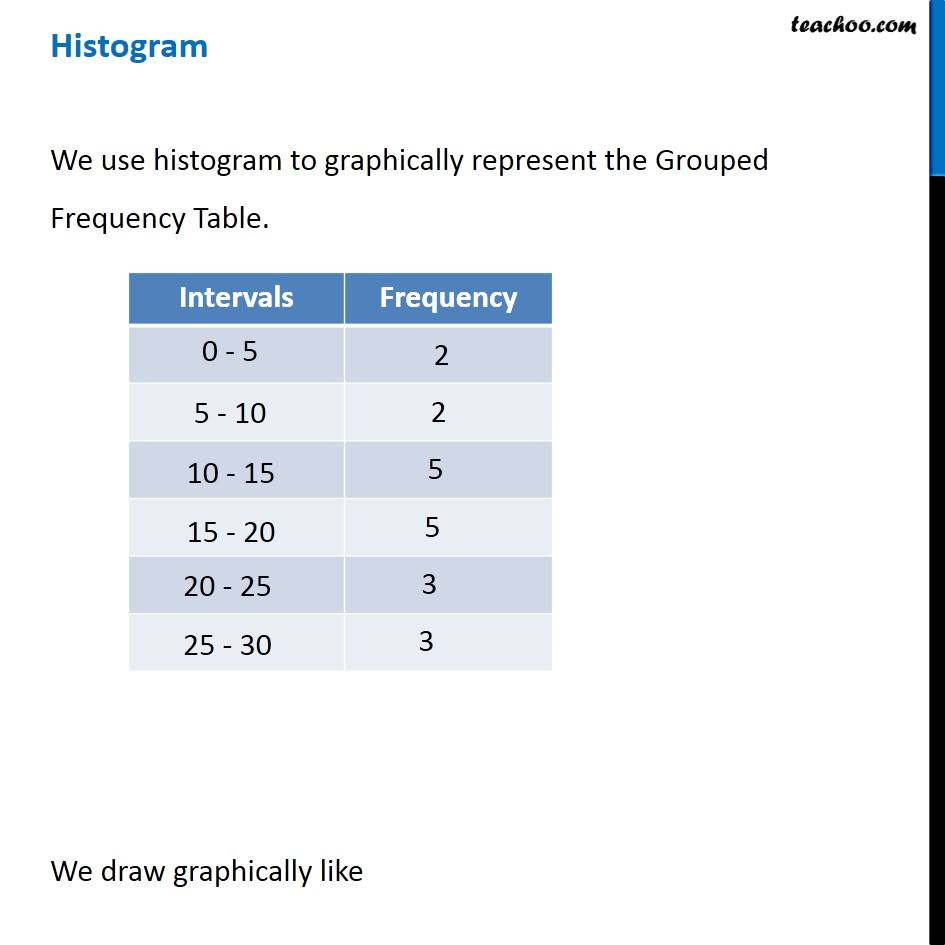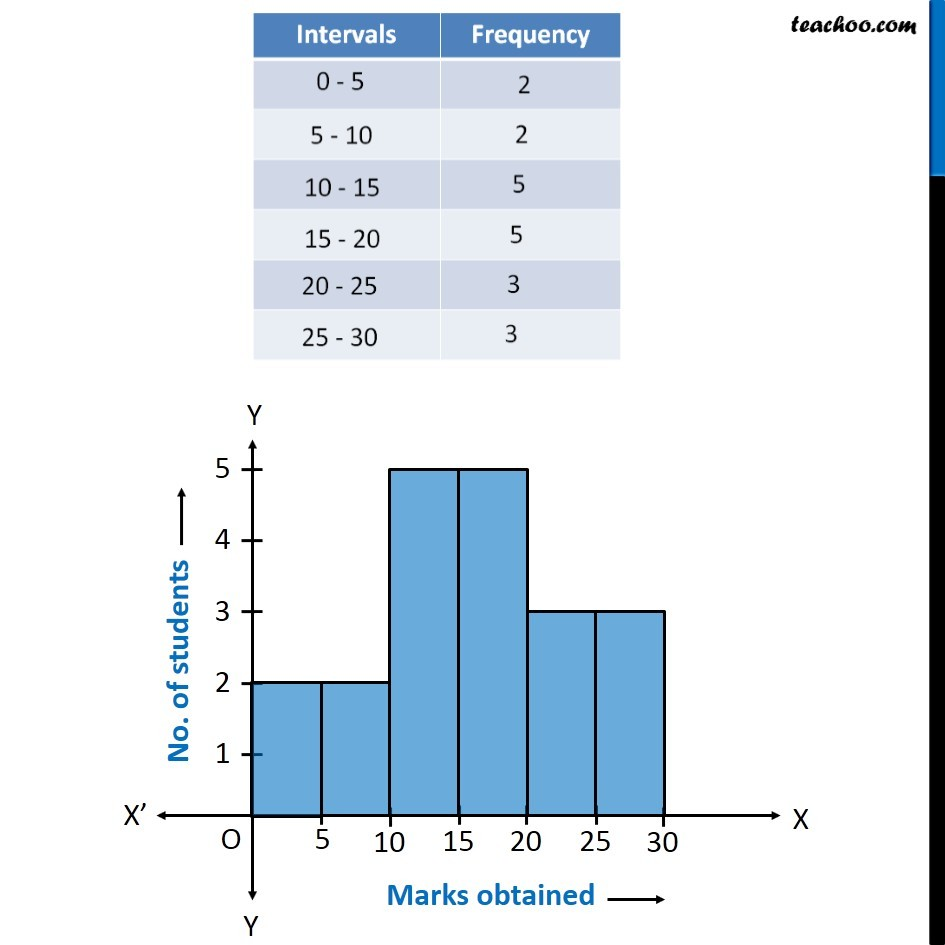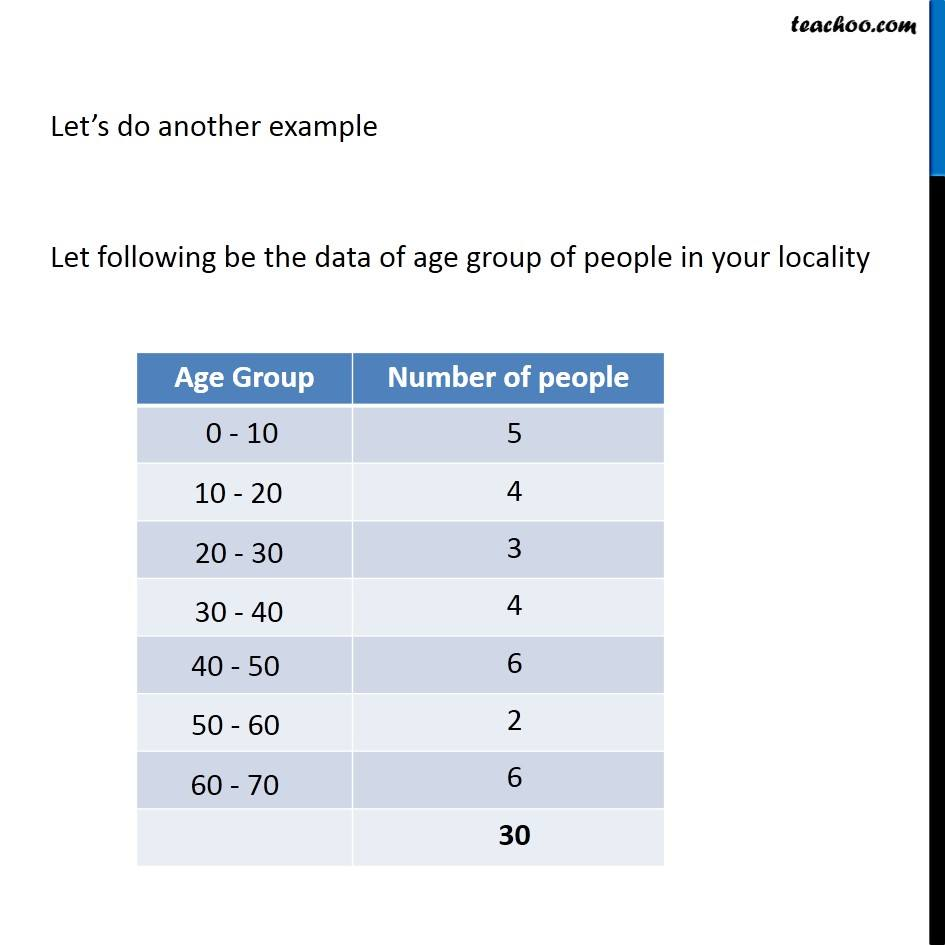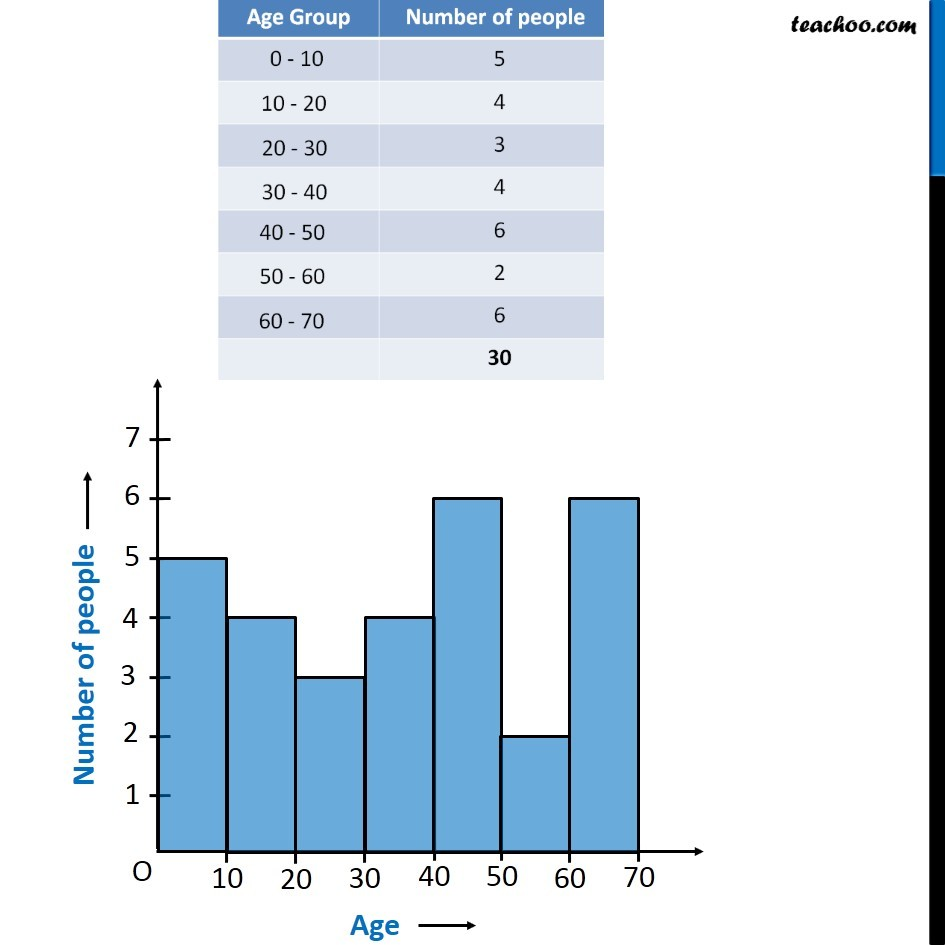1. Chapter 5 Class 8 Data Handling
2. Concept wise
3. Histogram

Transcript

We use histogram to graphically represent the Grouped Frequency Table. We draw graphically like Let’s do another example Let following be the data of age group of people in your locality Bar Graph In Bar Graph Bars have equal space On the y-axis, we have numbers & on the x-axis, we have data which can be anything. Histogram In Histogram Bars are fixed On the y-axis, we have numbers & on the x-axis, we have data which is continuous & will always be number. Bars chart can also be drawn horizontally. Histogram cannot be drawn horizontally.

Histogram

Chapter 5 Class 8 Data Handling
Concept wise